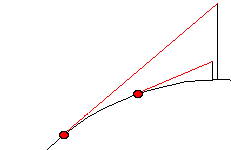# When I stand at the water's edge and look out over the ocean, how far away is the horizon?One of the funniest things about the ocean is the fact that its surface is curved. We tend to think about water forming large flat sheets, but the surface of a large body of water is not actually flat at all -- it follows the curvature of the Earth.

Because of the curvature of our planet, the distance between you and the horizon when you look out over the ocean depends on your height above the surface of the water. The following diagram shows you how the distance to the horizon can change depending on the height of the observer:

So the distance to the horizon depends on the height of your eyes above the water. If your eyes are 8 inches (20 cm) above the water, the distance of the horizon is about 1 mile (1.6 km) away. A rough formula for calculating the distance to the horizon is:

SquareRoot(height above surface / 0.5736) = distance to horizon

where "height above surface" is in feet and "distance to horizon" is in miles. If you are 6 feet tall and standing right at the water's edge, then your eyes are about 5.5 feet above the surface. The distance to the horizon is:

SquareRoot(5.5 / 0.5736) = 3 miles

In metric, the equivalent is:

SquareRoot(height above surface / 6.752) = distance to horizon

where "height above surface" is in centimeters and "distance to horizon" is in kilometers.

Originally Published: Apr 1, 2000

### How Far Is The Horizon FAQ

##### What is the horizon?
The horizon is the mid-way point between the Earth and the sky. It's where these two parts seem to meet.
##### How far can you see on the horizon?
If you are looking at the horizon from the ground, the horizon is approximately 5 kilometers from you.
##### How far is the horizon in miles?
The furthest distance to the horizon that the human eye can see varies slightly depending on a person’s height. In miles, the horizon is approximately 3.1 miles away.
##### How far can you see clouds on the horizon?
If you’re standing on level ground, in clear weather and with ample light, you can see clouds on the horizon from about 66 kilometers away.
##### How do you calculate the distance to the horizon?
The formula to calculate the distance from you to the horizon is 1.17 times the square root of the height from the ground to your eyes.